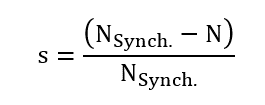# Inputs

## 1. Standard inputs

1. Line-line voltage, rms

The line-line voltage supplied to the machine: “Line-line voltage, rms” ( Line-line voltage, rms value ) must be provided.

2. Slip or Speed mode

There are two usual parameters to define the working point. It can be defined by the slip “ Slip ” or by the rotor speed “ Speed ”.

3. Slip

If the “Definition mode” is “ Slip ”, the value of the machine’s slip must be provided, and the corresponding speed is deduced.

The slip “s” in the following relations) corresponds to the relative difference between the synchronous speed (N Synch. ) and rotor speed N:4. Speed

If the “Definition mode” is “ Speed ”, the targeted rotor speed must be provided, and the resulting slip is deduced.

5. Power supply frequency

The power supply frequency corresponds to the electrical frequency of the stator magnetic field.

6. Represented coil conductors

In transient application, it is possible to export a project into Flux environment where the elementary wires will be modeled with solid conductors. The geometry, the meshing and the corresponding electric circuit will be defined to well represent them.

One choice is possible:

• All phases”: The elementary wires will be represented into all the phases

The list of advanced inputs dedicated to this export are listed below.

For more details please refer to the section 3.7.4 - List of generic advanced inputs.

1. Number of computations per electrical period

The default value is equal to 50. The minimum allowed value is 13.

2. Number of computed electrical periods

The default value is equal to 2. The minimum allowed value is 1 and the maximum value is equal to 10.

3. Rotor initial position
4. Mesh order

The default level is second order mesh.

5. Airgap mesh coefficient

Airgap mesh coefficient is set to 1.5 by default.